# Altitudes And Medians Of A Triangle

##### Class 8th Mathematics (new) MHB Solution

###### Practice Set 4.1
Question 1.

In ∆LMN, ...... is an altitude and ...... is a median. (write the names of appropriate segments.)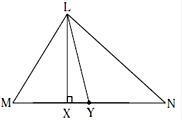In ∆LMN, LX is an altitude (because it makes a 90° angle on the base where it falls) and LY is a median (because it divides the base into two equal halves i.e., MY = NY).

Question 2.

Draw an acute-angled ∆PQR. Draw all of its altitudes. Name the point of concurrence as ‘O’.

To draw altitudes of a triangle:

i. Draw an acute-angled ∆PQR.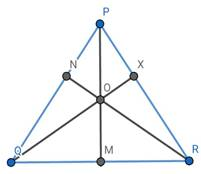ii. Draw a perpendicular from vertex P on the side QR using a set - square. Name the point where it meets side QR as M. Seg PM is an altitude on side QR.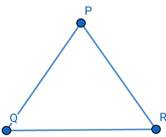iii. Considering side PR as a base, draw an altitude QX on side XZ. Seg QX is an altitude on side PR.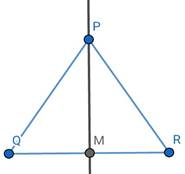iv. Consider side PQ as a base, draw an altitude RN on seg PQ. Seg RN is an altitude on side PQ.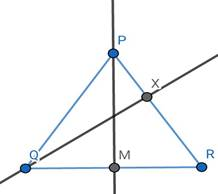Hence,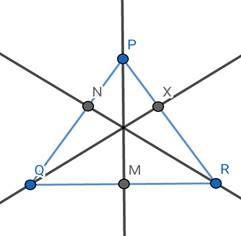Seg PM, seg QO, seg RN are the altitudes of ∆PQR. The point of concurrence i.e., the orthocentre is denoted by the point O.

Question 3.

Draw an obtuse-angled ∆STV. Draw its medians and show the centroid.

To draw an obtuse-angled ∆STV.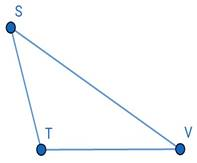i. Draw a base line of any length, mark it TV. At T draw an obtuse angle mark that line point S. Join S and V points. ΔSTV thus formed is an obtuse angled triangle.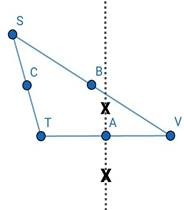ii. Find the mid-point A of side TV, by constructing the perpendicular bisector of the line segment TV. Draw AS.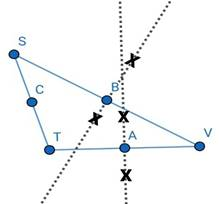iii. Find the mid-point B of side SV, by constructing the perpendicular bisector of the line segment SV. Draw seg BT.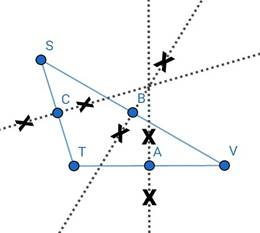iv. Find the mid-point C of side ST, by constructing the perpendicular bisector of the line segment ST. Draw seg CV.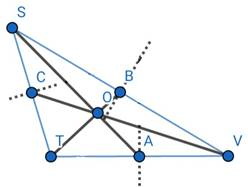Seg AS, seg BT and seg CV are medians of ∆STV.

Their point of concurrence is denoted by O.

Question 4.

Draw an obtuse-angled ∆LMN. Draw its altitudes and denote the orthocentre by ‘O’.

To draw an obtuse-angled ∆LMN.

i. Draw a base line of any length, mark it MN. At M draw an obtuse angle mark that line point L. Join L and N points. ΔLMN thus formed is an obtuse angled triangle.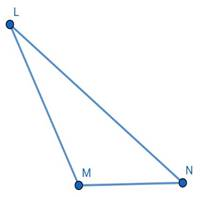ii. To draw an altitude from vertex L, extend side MN of the triangle from point M with a dashed line, as shown in the figure, and then draw the perpendicular lines from M.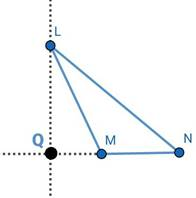iii. Considering side LN as a base, draw an altitude MP on side LN. Seg MP is an altitude on side LN.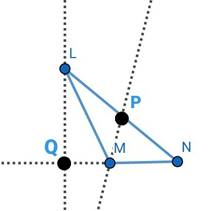iv. To draw altitude from vertex N, extend side LM of the triangle from point M with dashed line, as shown in the figure, and then draw the perpendicular line from vertex N.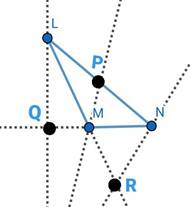v. Now for the orthocentre, as all the altitudes do not intersect we'll have to extend them so that they can meet giving us an orthocentre of the triangle.

vi. Hence, extend the altitude LQ, from point Q ; MP from point M, and NR from point R.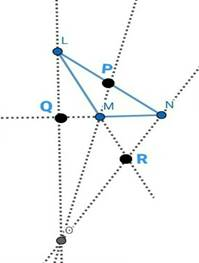vii. The ortho centre of the Obtuse triangle lies outside the triangle.

viii. The point O denotes the orthocentre of the obtuse-angled ∆LMN.

Question 5.

Draw a right angled ∆XYZ. Draw its medians and show their point of concurrence by G.

To draw an right angled ∆XYZ.

i. Draw a base line of any length, mark it YZ. At Y draw a right angle mark that line point X. Join X and Z points. ΔXYZ thus formed is right angled triangle.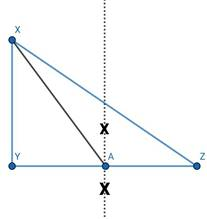ii. Find the mid-point A of side YZ, by constructing the perpendicular bisector of the line segment YZ. Draw AX.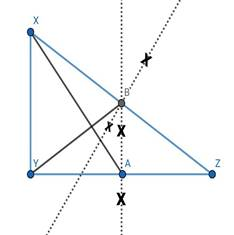iii. Find the mid-point B of side XZ, by constructing the perpendicular bisector of the line segment XZ. Draw seg BY.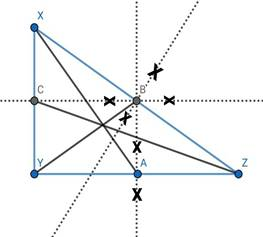iv. Find the mid-point C of side XY, by constructing the perpendicular bisector of the line segment XY. Draw seg CZ.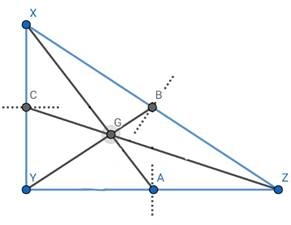Seg AX, seg BY and seg CZ are medians of ∆XYZ.

Their point of concurrence is denoted by G.

Question 6.

Draw an isosceles triangle. Draw all of its medians and altitudes. Write your observation about their points of concurrence.

i. Draw an isosceles triangle and name it as PQR.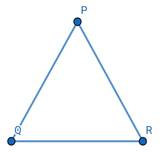An isosceles triangle is that triangle whose base is the side which is not equal to the other two sides or An isosceles triangle is a triangle which has two equal sides.

ii. Now, mark the mid-point i.e., A, B, C, of all the sides of the triangle and join it with the opposite vertex i.e., P, Q, R. The line segment i.e., PA, QB, RC hence found are the median of the triangle.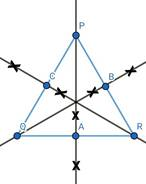iii. Mark the point of concurrence as 'O'.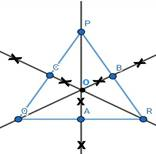iv. Again, draw perpendicular line segment from each vertex.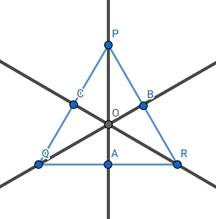v. Mark the point of concurrence X.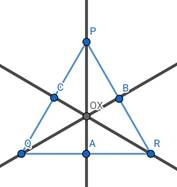Here we see that both the point of concurrence of medians and altitudes coincides.

In the case of isosceles triangle, the two sides that are equal meet at a vertex, that lies directly above the midpoint of the base. Because of this, the altitude that runs from P to the base intersects the base at its midpoint, making it the median from P to the base as well, which is same for the other two sides also.

Therefore, in an isosceles triangle, the altitude and median are the same line segment, which is shown through the bold line in the above-given figure.

Question 7.

Fill in the blanks.

Point G is the centroid of ∆ABC.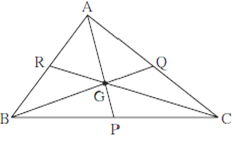(1) If l(RG) = 2.5 then l(GC) = ......

(2) If l(BG) = 6 then l(BQ) = ......

(3) If l(AP) = 6 then l(AG) = ..... and l(GP) = .....

1) If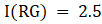then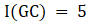, as we know that the centroid divides each median in the ratio 2:1.

Hence,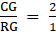GC/2.5 = 2/1

Cross Multiplying we get,

GC × 1 = 2 × 2.5

Therefore, I(GC) = 5

2) If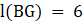then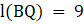, as we know that the centroid divides each median in the ratio 2:1.

Now,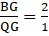6/QG = 2/1

6 × 1 = 2 × QG

6 = 2 × QG

6/2 = QG

Hence, I(QG) = 3.

Since we have to find I(BQ), and from the figure it can be seen that,

(BQ) = I(BG) + I(QG)

Therefore, I(BQ) = 6 + 3

I(BQ) = 9.

3) If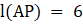then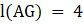and l(GP) = 2, as we know that the centroid divides each median in the ratio 2:1 --------(i)

Here both I(AG) and I(GP) are unknown so,

Let I(AG), I(GP) be 2x and x respectively, from equation (i)

Since, I(AP) = I(AG) + I(GP)

6 = 2x + x

6 = 3x

6/3 = x

x = 2.

Therefore, I(AG) = 2x = 2×2 = 4.

I(GP) = x = 2.

## PDF FILE TO YOUR EMAIL IMMEDIATELY PURCHASE NOTES & PAPER SOLUTION. @ Rs. 50/- each (GST extra)

SUBJECTS

HINDI ENTIRE PAPER SOLUTION

MARATHI PAPER SOLUTION
SSC MATHS I PAPER SOLUTION
SSC MATHS II PAPER SOLUTION
SSC SCIENCE I PAPER SOLUTION
SSC SCIENCE II PAPER SOLUTION
SSC ENGLISH PAPER SOLUTION
SSC & HSC ENGLISH WRITING SKILL
HSC ACCOUNTS NOTES
HSC OCM NOTES
HSC ECONOMICS NOTES
HSC SECRETARIAL PRACTICE NOTES

2019 Board Paper Solution

HSC ENGLISH SET A 2019 21st February, 2019

HSC ENGLISH SET B 2019 21st February, 2019

HSC ENGLISH SET C 2019 21st February, 2019

HSC ENGLISH SET D 2019 21st February, 2019

SECRETARIAL PRACTICE (S.P) 2019 25th February, 2019

HSC XII PHYSICS 2019 25th February, 2019

CHEMISTRY XII HSC SOLUTION 27th, February, 2019

OCM PAPER SOLUTION 2019 27th, February, 2019

HSC MATHS PAPER SOLUTION COMMERCE, 2nd March, 2019

HSC MATHS PAPER SOLUTION SCIENCE 2nd, March, 2019

SSC ENGLISH STD 10 5TH MARCH, 2019.

HSC XII ACCOUNTS 2019 6th March, 2019

HSC XII BIOLOGY 2019 6TH March, 2019

HSC XII ECONOMICS 9Th March 2019

SSC Maths I March 2019 Solution 10th Standard11th, March, 2019

SSC MATHS II MARCH 2019 SOLUTION 10TH STD.13th March, 2019

SSC SCIENCE I MARCH 2019 SOLUTION 10TH STD. 15th March, 2019.

SSC SCIENCE II MARCH 2019 SOLUTION 10TH STD. 18th March, 2019.

SSC SOCIAL SCIENCE I MARCH 2019 SOLUTION20th March, 2019

SSC SOCIAL SCIENCE II MARCH 2019 SOLUTION, 22nd March, 2019

XII CBSE - BOARD - MARCH - 2019 ENGLISH - QP + SOLUTIONS, 2nd March, 2019

HSC Maharashtra Board Papers 2020

(Std 12th English Medium)

HSC ECONOMICS MARCH 2020

HSC OCM MARCH 2020

HSC ACCOUNTS MARCH 2020

HSC S.P. MARCH 2020

HSC ENGLISH MARCH 2020

HSC HINDI MARCH 2020

HSC MARATHI MARCH 2020

HSC MATHS MARCH 2020

SSC Maharashtra Board Papers 2020

(Std 10th English Medium)

English MARCH 2020

HindI MARCH 2020

Hindi (Composite) MARCH 2020

Marathi MARCH 2020

Mathematics (Paper 1) MARCH 2020

Mathematics (Paper 2) MARCH 2020

Sanskrit MARCH 2020

Important-formula

THANKS